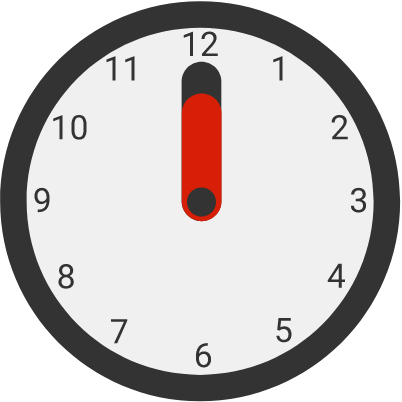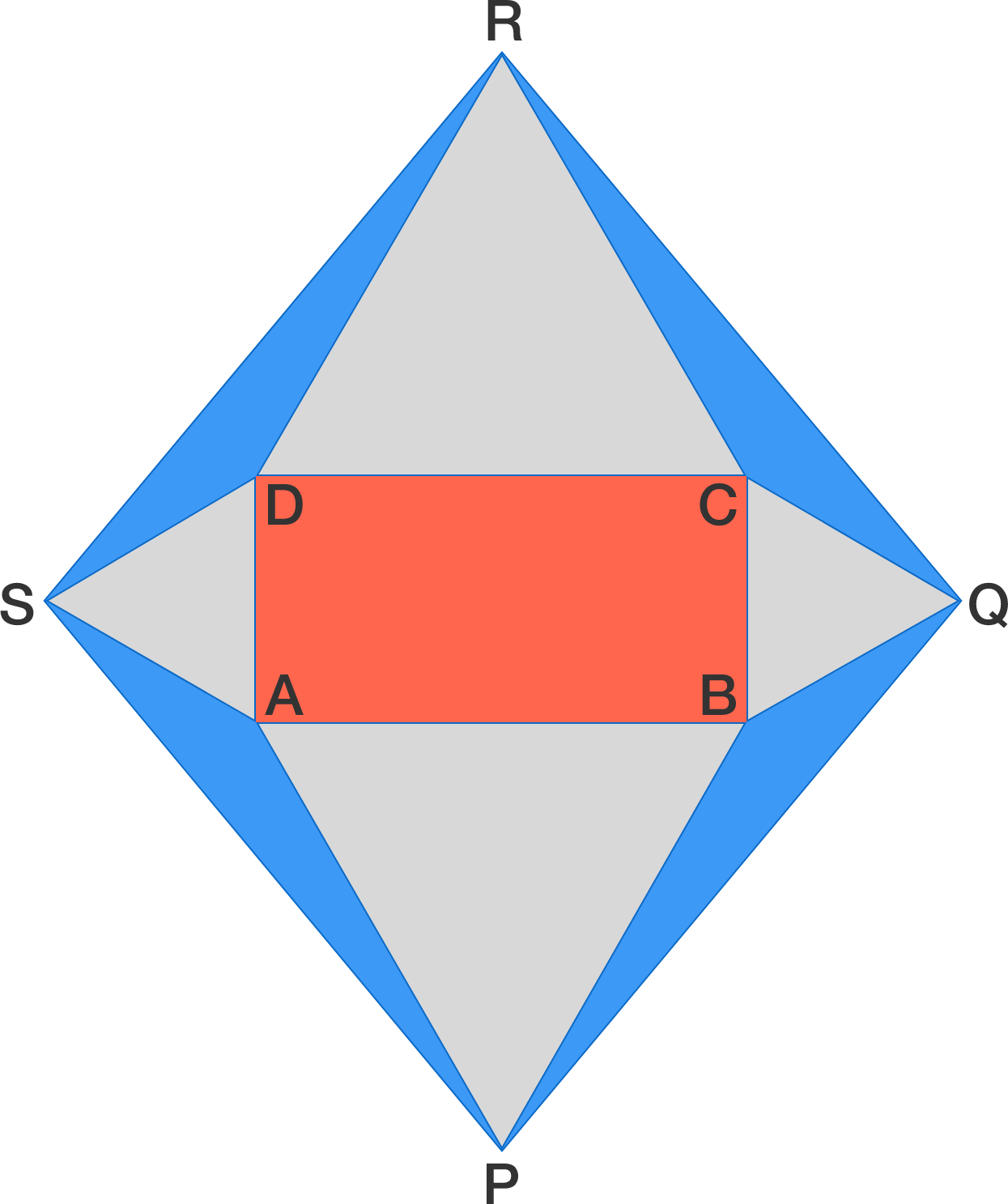# 2017-05-29 IntermediateHow many times do the minute and hour hands of a clock perfectly overlap in 24 hours, from 7 a.m. to 7 a.m. the next day?


Clarification: It is a standard analog clock where both hands move continuously.

$\large 17^{7} - 17$ Is the above number a multiple of 36?In the diagram above, $ABCD$ is a rectangle and triangles $ABP, BCQ, CDR, DAS$ are all equilateral. Which has a larger area, the red rectangle or the sum of the 4 blue triangles?

Sharky and Ivan are playing a strange game, with Sharky going first.

They start with a heap of 420 coins. They then take turns to remove some of the coins using the two rules below.

Given that the number of coins in the pile is $k,$ you may either

1. remove the largest power of 2 less than $k,$ if $k$ is not a power of 2, or
2. remove half the coins only if $k$ is even.

If a person cannot make a legal move, they lose. Who has the winning strategy?

Alice and Bob saw this matrix with infinite rows and columns:

$\begin{pmatrix} 1 & 0 & 0 & 0 & 0 & \cdots \\ -1 & 1 & 0 & 0 & 0 & \cdots \\ 0 & -1 & 1 & 0 & 0 & \cdots \\ 0 & 0 & -1 & 1 & 0 & \cdots\\ 0 & 0 & 0 & -1 & 1 & \cdots\\ \vdots & \vdots & \vdots & \vdots & \vdots & \ddots \end{pmatrix}.$

They wanted to sum up all the elements in it.

Alice: "The sum of each column is 0, so the sum of all the numbers must be 0."
Bob: "The sum of all the rows except the first one is 0. The first row adds up to 1. Hence, the sum of all the numbers is 1."

Who is correct?

×

Problem Loading...

Note Loading...

Set Loading...